# 从零开始学习zk-SNARK(四)—多项式的约束

## 导读

even@安比实验室: 上一篇文章中我们学习了如何将程序转换为多项式进行证明。到这里似乎已经有点晕了，本文将对协议执行进一步的约束，并对协议展开优化。

【本系列文章】

1. 多项式的性质与证明
2. 多项式的非交互式零知识证明
3. 从程序到多项式的构造
4. 多项式的约束
5. Pinocchio 协议

## 结构性质

### 静态系数

<font style=”color:green”>2a</font> × <font style=”color:blue”>1b</font> = <font style=”color:red”>3r</font> <font style=”color:green”>-3a</font> × <font style=”color:blue”>1b</font> = <font style=”color:red”>12r</font>

Algorithm 2: Constant coefficients
—————————————————————————————————————————————————————

function calc(w, a, b)
if w then
return 3a × b
else
return 5a × 2b
end if
end function

### 没有成本的做加法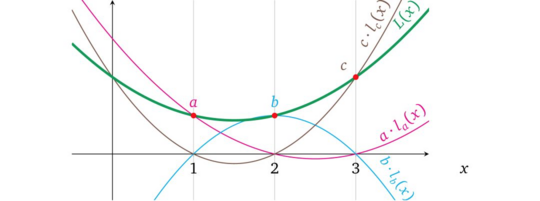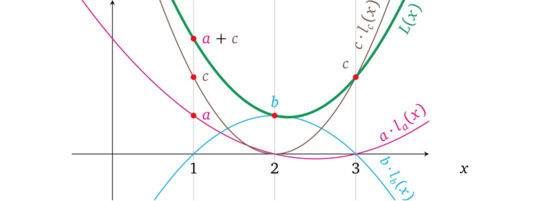<font style=”color:green”>(c<sub>1,a</sub>+c<sub>1,b</sub>+…)</font> × <font style=”color:blue”>(c<sub>r,a</sub>+c<sub>r,b</sub>+…)</font> = <font style=”color:red”>(c<sub>o,a</sub>+c<sub>o,b</sub>+…)</font>

$c{l,i}\in c{l,1},c{l,2},…,c{l,n},$ $c{r,i}\in c{r,1},c{r,2},…,c{r,n},$ $c{o,i}\in c{o,1},c{o,i},…,c{o,n},$

<font style=”color:green”>$\sum{i=1}^n c{l,i}\cdot vi$</font> × <font style=”color:blue”>$\sum{i=1}^n c_{l,i}\cdot vi$</font> = <font style=”color:red”>$\sum{i=1}^n c_{o,i}\cdot v_i$</font>

### 加法，减法和除法

@Maksym（作者）：因为我们的结构中对于每一个操作数我们既需要常量系数也需要变量 (c ⋅ v) ，1 这个值可以表示为 c₁ ⋅ v₁，其中 c₁ = 1 可以被“硬编码”到对应的多项式中， v₁ 是一个变量可以给它分配任何值，那么我们就必须通过一些约束来限制 v₁ 的值，这个在后面的章节中将会讲到。

<font style=”color:green”>(a+ -1·b)</font> × <font style=”color:blue”>1</font> =<font style=”color:red”>r</font>

$\frac{factor}{divisor}=result$

<front style=”color:green”>b</front> × <front style=”color:blue”>r</front> = <front style=”color:red”>a</front>

@Maksym（作者）：运算的结构也称为 “约束” ，因为多项式结构代表的运算，并非是为了计算出结果，而是在 prover 已经知晓的变量赋值的情况下，检验这个运算的过程是否正确。换句话说，即约束 prover 必须提供一致的值，无论这些值是什么。 所有的算术计算（加减乘除）都已经有了，于是运算结构不再需要修改。

## 计算示例

$w \times (a \times b) + (1-w) \times (a+b)=v$

3： <front style=”color:green”>1·w</front> × <front style=”color:blue”>1·w</front> = <front style=”color:red”>1·w</front>

<front style=”color:green”>$L(x)=a \cdot l_a(x)+w \cdot l_w(x)$</front> <front style=”color:blue”>$R(x)=m \cdot r_m(x)+a \cdot r_a(x)+b \cdot r_b(x)$</front> <front style=”color:red”>$O(x)=m \cdot o_m(x)+v \cdot o_v(x)+a \cdot o_a(x)+b \cdot o_b(x)$</front>

<front style=”color:green”>$l_w(1)$</front>=0;<front style=”color:green”>$l_w(2)$</front>= 1;<front style=”color:green”>$l_w(3)$</front>=1;<front style=”color:blue”>$r_a(1)$</front>=0;<front style=”color:blue”>$r_a(2)$</front>=-1;<front style=”color:blue”>$r_a(3)$</front>=0;<front style=”color:red”>$o_v(1)$</front>=0;<front style=”color:red”>$o_v(2)$</front>=1;<front style=”color:red”>$o_v(3)$</front>=0;

$\ \ \ \ \ \ \ \ \ \ \ \ \ \ \ \ \ \ \ \ \ \ \ \ \ \ \ \ \ \ \ \ \ \ \ \ \ \ \ \$<front style=”color:blue”>$r_b(1)$</front>=1;<front style=”color:blue”>$r_b(2)$</front>= -1;<front style=”color:blue”>$r_b(3)$</front>=0;<front style=”color:red”>$o_a(1)$</front>=0;<front style=”color:red”>$o_a(2)$</front>= -1;<front style=”color:red”>$o_a(3)$</front>=0;

$\ \ \ \ \ \ \ \ \ \ \ \ \ \ \ \ \ \ \ \ \ \ \ \ \ \ \ \ \ \ \ \ \ \ \ \ \ \ \ \$<front style=”color:blue”>$r_w(1)$</front>=1;<front style=”color:blue”>$r_w(2)$</front>= 0;<front style=”color:blue”>$r_w(3)$</front>=0;<front style=”color:red”>$o_b(1)$</front>=0;<front style=”color:red”>$o_b(2)$</front>= -1;<front style=”color:red”>$o_b(3)$</front>=0;

$\ \ \ \ \ \ \ \ \ \ \ \ \ \ \ \ \ \ \ \ \ \ \ \ \ \ \ \ \ \ \ \ \ \ \ \ \ \ \ \ \ \ \ \ \ \ \ \ \ \ \ \ \ \ \ \ \ \ \ \ \ \ \ \ \ \ \ \ \ \ \ \ \ \ \ \ \ \ \ \ \ \ \ \$<front style=”color:red”>$o_w(1)$</front>=0;<front style=”color:red”>$o_w(2)$</front>= 0;<front style=”color:red”>$o_w(3)$</front>=1;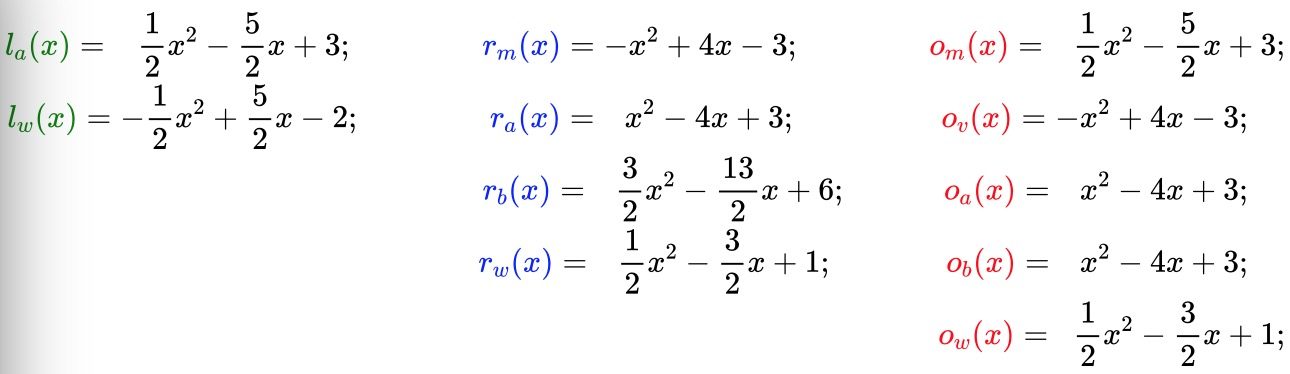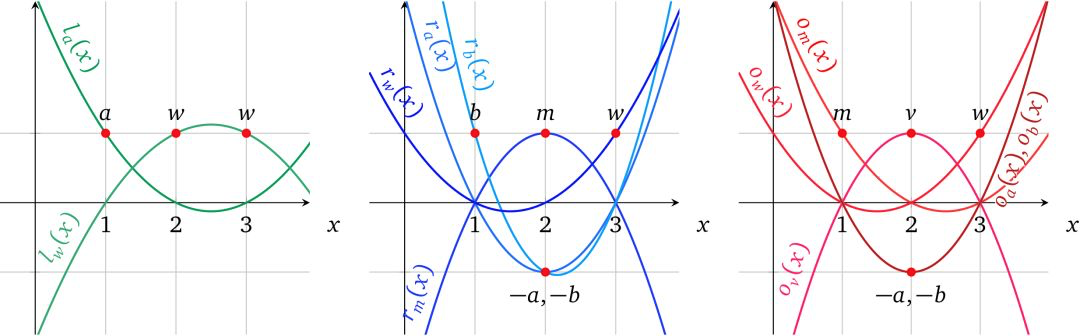m=a × b =6 v = w(m-a-b)+a+b=6

<front style=”color:green”>$L(x)=e \cdot l_a(x) + 1 \cdot l_w(x)$</front>=$x^2 – 5x +7$

<front style=”color:blue”>$R(x)=6 \cdot r_m(x) + 3 \cdot r_a(x)+2 \cdot r_b(x) + 1 \cdot r_w(x)$</front>=$\frac12 x^2-2\frac12x+4$

<front style=”color:red”>$O(x)=6 \cdot o_m(x) + 6 \cdot o_v(x)+3 \cdot o_a(x) + 2 \cdot o_b(x)+ 1 \cdot o_w(x)$</front>$=2\frac12 x^2-12\frac12x+16$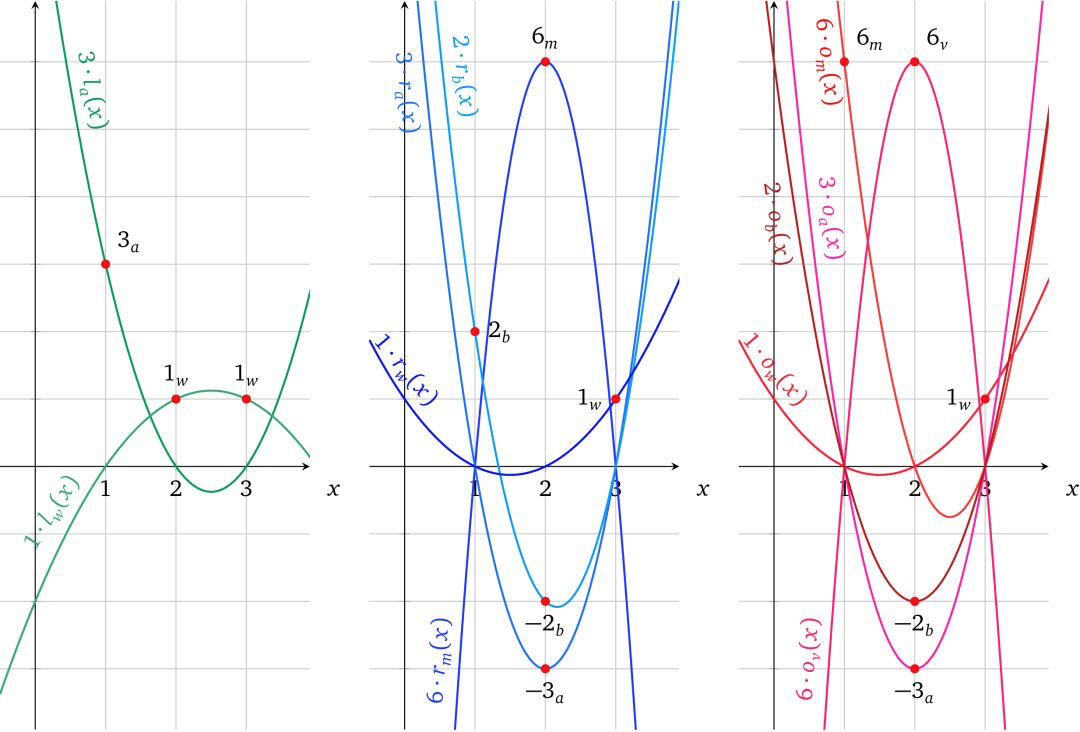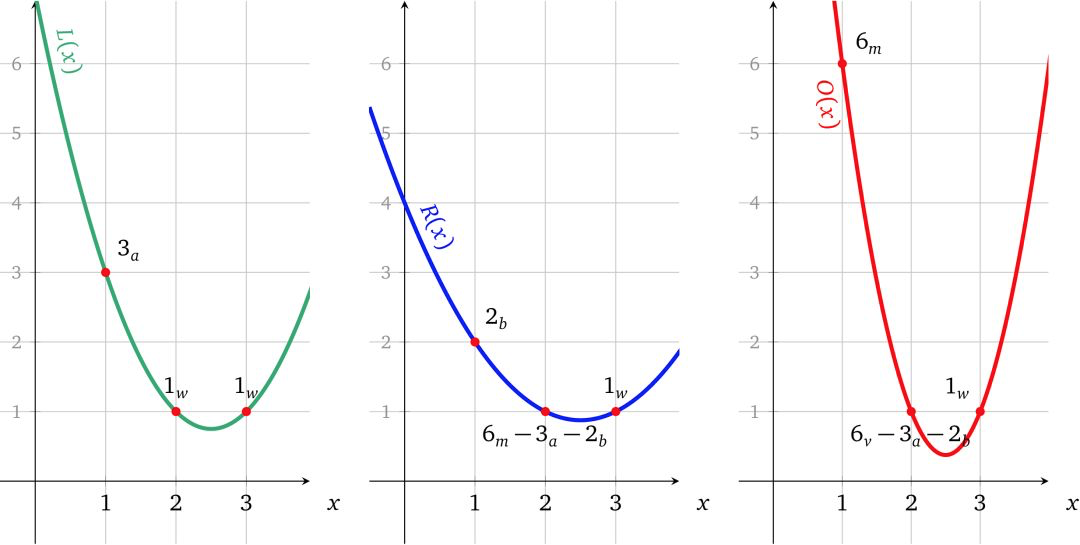$h(x)=\frac{L(x)\times R(x)-O(x)}{t(x)}$ $=\frac{\frac12x^4-5x^3+\frac{35}2x^2-25x+12}{(x-1)(x-2)(x-3)}=\frac12x-2$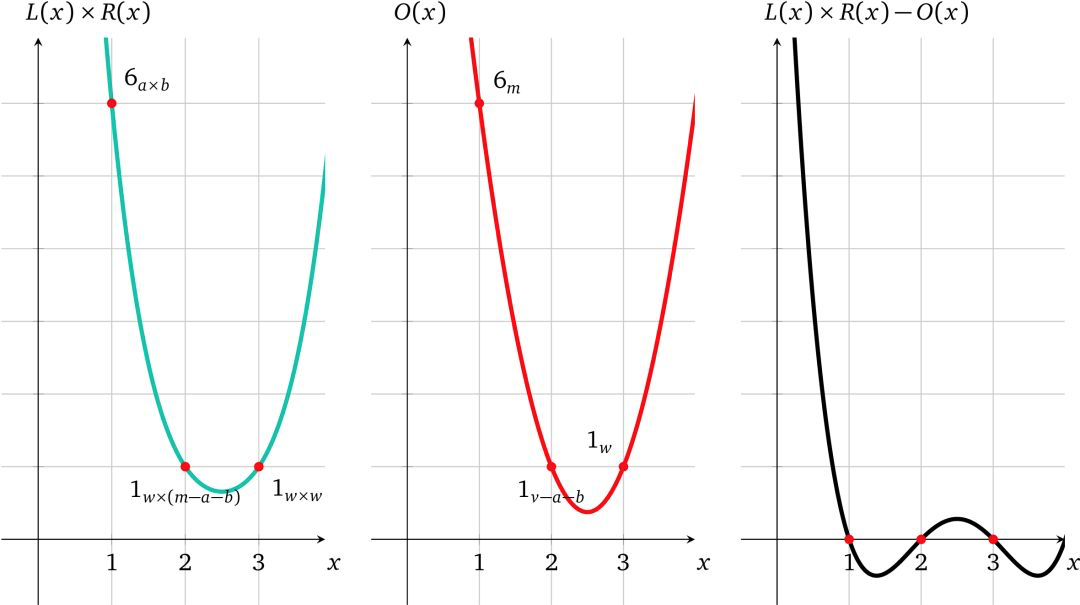## 可验证计算协议

• Setup

• 为左操作数$\left{l_i(x)\right}i\in \left{1,..,n\right}$ 构造变量多项式 然后对于所有$j\in \left{1,..,d\right}$ 的运算都算出其对应的系数，即$li(j)=c{L,i,j}$ ，对右操作数和输出也做同样的事情。

• 随机抽取 s，α

• 计算 t(x)= (x-1)(x-2)…(x-d) 及其结果$g^{t(s)}$

• 计算 proving key： $(\left{ g^{s^k} \right}k \in [d],\left{ g^{l_i(s)}, g^{r_i(s)}, g^{o_i(s)}, g^{\alpha l_i(s)}, g^{\alpha r_i(s)}, g^{\alpha o_i(s)} \right} i \in \left{ 1,..,n \right})$

• 计算 verification key： $(g^{t(s),g^\alpha})$

• Proving

• 计算函数 f(*) 以此计算相应的变量 $\left{ v_i\right} i \in \left{ 1,..,n\right}$

• 计算$h(x)=\frac{L(x)\times R(x)-O(x)}{t(x)}$ ，其中$L(x)=\sum^n_{i=1} v_i\cdot l_i(x),R(x),O(x)$与之相似

• 给变量赋值并对操作数多项式求和： $g^{L(s)}=(g^{l_1(s)})^{v_1}…(g^{l_n(s)})^{vn},g^{R(s)}$ $=\prod^n{i=1}(g^{r_i(s)})^{vi},g^{O(s)}=\prod^n{i=1}(g^{o_i(s)})^{v_i}$

• 对变换后的多项式赋值： $g^{\alpha L(s)}=\prod^n_{i=1}(g^{\alpha l_i(s)})^{vi},g^{\alpha R(s)}$ $=\prod^n{i=1}(g^{\alpha r_i(s)})^{vi},g^{\alpha O(s)}=\prod^n{i=1}(g\alpha o_i(s))^{v_i}$

• 使用 s 的幂加密值:$\left{g^{s^k} \right}k\in[d]$ 计算加密值 $g^{h(s)}$

• 生成证明：$\left{g^{L(s)},g^{R(s)},g^{O(s)},g^{\alpha L(s)},g^{\alpha R(s)},g^{\alpha O(s)},g^{h(s)} \right}$

• Verification

• 解析证明为 $\left{g^L,g^R,g^O,g^{L’},g^{R’},g^{O’},g^h \right}$

• 检查可变多项式约束：$e(g^L,g^\alpha)=e(g^{L’},g),e(g^R,g^\alpha)=e(g^{R’},g),e(g^O,g^\alpha)=e(g^{O’},g)$

• 验证计算有效性：$e(g^L,g^R)=e(g^t,g^h)\cdot e(g^O,g)$

### 操作数和输出的不可交换性

• 使用其它的操作数中的可变多项式，即 L′(s) = o₁(s) + r₁(s) + r₁(s) + …

• 完全交换 操作数多项式， 也就是把 O(s) 和 L(s) 换成 O(s) × R(s) = L(s)

• 复用相同的操作数多项式，即 L(s) × L(s) = O(s)

• Setup …

• 选择随机数$\alpha_l,\alpha_r,\alpha_o$ 来代替 α

• 计算其对应的“变换” $\left{ g^{\alpha_ll_i(s)},g^{\alpha_rr_i(s)},g^{\alpha_oo_i(s)} \right}i\in \left{ 1…n\right}$

• proving key：$(\left{ g^{s^k}\right}k\in[d]\left{ g^{l_i(s)},g^{r_i(s)},g^{o_i(s)},g^{\alpha_ll_i(s)},g^{\alpha_rr_i(s)},g^{\alpha_oo_i(s)}\right}i\in \left{ 1…n\right})$

• verfication key：$(g^{t(s)},g^{\alpha _l},g^{\alpha _r},g^{\alpha _o})$

• Proving …

• 为 “变换”的多项式赋值： $g^{\alphalL(s)}=\prod^n{i=1}(g^{\alpha_ll_i(s)})^{v_i}$, $g^{\alphalR(s)}=\prod^n{i=1}(g^{\alpha_rr_i(s)})^{v_i}$, $g^{\alphalO(s)}=\prod^n{i=1}(g^{\alpha_oo_i(s)})^{v_i}$

• 设置证明：$(g^{L(s)},g^{R(s)},g^{O(s)},g^{\alpha_lL(s)},g^{\alpha_rR(s)},g^{\alpha_oO(s)},g^{h(s)})$

• Verification …

• 可变多项式约束验证： $e(g^L,g^{\alpha_l})=e(g^{L’},g),e(g^R,g^{\alpha_r})=e(g^{R’},g),e(g^O,g^{\alpha_o})=e(g^{O’},g)$

### 跨操作数的变量一致性

• Setup

• … 随机数 $\beta_l,\beta_r,\beta_o$

• 对变量一致性多项式 进行计算，加密并添加到 proving key 中： $g^{ \beta_l l_i(s)+\beta_r r_i(s)+\beta_o o_i(s) } i \in \left{ 1,…,n \right}$

• 对 $\beta_s$加密并将其加到 verification key 中：$( g^{\beta_l},g^{\beta_r},g^{\beta_o} )$

• Proving

• …将变量值赋给变量一致性多项式： $g^{z_i(s)}=( g^{ \beta_l l_i(s)+\beta_r r_i(s)+\beta_o o_i(s) } )^{v_i}\ \ for \ \ i \in \left{ 1,…,n \right}$

• 增加分配的多项式到加密空间中: $g^{Z(s)}=\prod^n_{i=1} g^{z_i(s)} =g^{ \beta_lL(s)+\beta_rR(s)+\beta_oO(s) }$

• 再在证明中加入：$g^{Z(s)}$

• Verification

• …校验提供的操作数多项式 和 “校验和”多项式之间的一致性： $e(g^L,g^{\beta_l}) \cdot e(g^R,g^{\beta_r}) \cdot e(g^O,g^{\beta_o}) = e(g^Z,g)$

### 变量非延展性和变量一致性多项式

#### 变量多项式的延展性

• …Proving：

• 用分配不成比例的变量 a 来建立左操作数多项式： $L(x)=a \cdot l_a(x)+1$

• 按照常规的方式构造右操作数多项式和输出多项式： $R(x) = r_1(x),O(x) = b \cdot o_b(x) + c \cdot o_c(x)$

• 计算除数：$h(x) = \frac{L(x) \cdot R(x) – O(x)}{t(x)}$

• 计算加密值：$g^{L(s)} = (g^{l_\alpha (s)})^\alpha \cdot g^1$，并按照常规方式计算 $g^{R(s)},g^{O(s)}$

• 计算 α-变换的加密值：$g^{\alpha L(s)} = ( g^{ \alpha l_\alpha (s) } )^ \alpha \cdot g^\alpha$ ，并按照常规方式计算 $g^{\alpha R(s)},g^{\alpha O(s)}$

• 计算变量一致性多项式：$g^{Z(s)} = \prod_{i \in 1,a,b,c} (g^{ \beta_ll_i(s)+\beta_rr_i(s)+\beta_oo_i(s) })^i \cdot g^{\beta_l} = g^{ \beta_l(L(s)+1)+\beta_rR_i(s)+\beta_oO_i(s) }$ ，其中下标 i 代表对应变量的符号；指数 i 代表变量的值；以及未定义的变量多项式的值为 0。

• 设置证明： $( g^{L(s)},g^{R(s)},g^{O(s)},g^{\alpha _lL(s)},g^{\alpha _rR(s)},g^{\alpha _oO(s)},g^{Z(s)},g^{h(s)} )$

• Verification：

• 变量多项式约束检查： $e(g^L,g^{\beta_l}) \cdot e(g^R,g^{\beta_r}) \cdot e(g^O,g^{\betao})$ $=e(g^Z,g) \implies e(g,g)^{ (\alpha \cdot l\alpha+1)\beta_l + R \beta_r + O \beta_o }$ $=e(g,g)^{ \beta_l(L+1)+\beta_rR+\beta_oO }$

• 有效计算检查：$e(g^L,g^R) = e(g^t,g^h) \cdot e(g^O,g)$

#### 变量一致性多项式的延展性

• Proving：

• …用 a=2 设置左操作数多项式：$L(x)=2l_a(x)+10L_b(x)$

• 用 a=5 设置右操作数多项式：$R(x)=2r_a(x)+3+10r_b(x)$

• 用 b=10 设置输出多项式：$O(x)=2o_a(x)+10o_b(x)$

• …计算加密值： $g^{L(s)}=( g^{l_a(s)} )^2 \cdot ( g^{l_b(s)} )^{10} = g^{2l_a(s)+10l_b(s)}$ $g^{R(s)}=( g^{r_a(s)} )^2 \cdot (g)^3 \cdot ( g^{r_b(s)} )^{10} = g^{2r_a(s)+10r_b(s)}$ $g^{O(s)}=( g^{O_a(s)} )^2 \cdot ( g^{O_b(s)} )^{10} = g^{2o_a(s)+10o_b(s)}$

• 计算变量一致性多项式： $g^{Z(s)}=( g^{ \beta_ll_a(s)+\beta_rr_a(s)+\beta_oo_a(s) } )^2 \cdot ( g^{\beta_r} )^3 \cdot ( g^{ \beta_ll_b(s)+\beta_rr_b(s)+\beta_oo_b(s) } )$ $=g^{ \beta_l( 2l_a(s)+10l_b(s) ) + \beta_r( 2r_a(s)+3+10r_b(s) ) + \beta_o( 2o_a(s)+3+10o_b(s) ) }$

• Verification：

• ……变量值的一致性检查，应满足： $e(g^L,g^{\beta_l}) \cdot e(g^R,g^{\beta_r}) \cdot e(g^O,g^{\beta_o}) = e(g^Z,g )$

#### 非延展性

• Setup：

• …随机数 $β_l，β_r，β_o，γ$

• …设置 verification key：$( …,g^{\beta_l \gamma},g^{\beta_r \gamma},g^{\beta_o \gamma},g^\gamma )$

• Proving：…

• Verification：

• …变量值一致性检查应满足： $e(g^L,g^{\beta_l\gamma}) \cdot e(g^R,g^{\beta_r\gamma}) \cdot e(g^O,g^{\beta_o\gamma}) =e(g^Z,g^\gamma)$

### 变量值一致性检查的优化

• …Proving：

• 用分配不成比例的变量 a 来建立左操作数多项式：$L(x)=a \cdot l_a(x)+1$

• 按照常规的方式构造右操作数多项式和输出多项式： $R(x)=r_1(x),O(x)=b \cdot o_b(x) +c \cdot o_c(x)$

• 计算除数：$\frac{L(x) \cdot R(x) -O(x)}{t(x)}$

• 计算加密值：$g^{L(s)}=( g^{l_a(s)} )^a \cdot g^1$ ，并按照常规方式计算 $g^R(s),g^{O(s)}$

• 计算 α-变换的加密值：$g^{\alpha L(s)} = ( g^{\alpha l_a(s)} )^a \cdot g^\alpha$ ，并按照常规方式计算 $g^{\alpha R(s)},g^{\alpha O(s)}$

• 计算变量一致性多项式： $g^{Z(s)} = \prod_{i \in 1,a,b,c} ( g^{ \beta_ll_i(s)+\beta_rr_i(s)+\beta_oo_i(s) } )^i \cdot g^{\beta_l}$ $= g^{ \beta_l(L(s)+1)+\beta_rR(s)+\beta_oO(s) }$，其中下标 i 代表对应变量的符号；指数 i 代表变量的值；以及未定义的变量多项式的值为 0。

• 设置证明： $( g^{L(s)},g^{R(s)},g^{O(s)},g^{\alpha_lL(s)},g^{\alpha_rR(s)},g^{\alpha_oO(s)},g^{Z(s)},g^{h(s)} )$

• Verification：

• 变量多项式约束检查： $e(g^L,g^{\beta_l}) \cdot e(g^R,g^{\beta_r}) \cdot e(g^O,g^{\beta_o}) = e(g^Z,g)$ $\implies e(g,g)^{ (a \cdot l_a +1)\beta_l +R\beta_r+O\beta_o } = e(g,g)^{ \beta_l(L +1)\beta_l +R\beta_r+O\beta_o }$

• 有效计算检查：$e(g^L,g^R)=e(g^t,g^h) \cdot e(g^O,g)$

• 协议中是如何增加可变系数的和如何做加减乘除运算的
• 协议如何保证操作数和输出的不可替代性
• 协议如何保证跨操作数的可变一致性
• 协议如何处理非延展性变量和变量一致性
• 协议中变量值一致性检查优化

https://medium.com/@imolfar/why-and-how-zk-snark-works-5-variable-polynomials-3b4e06859e30

https://medium.com/@imolfar/why-and-how-zk-snark-works-6-verifiable-computation-protocol-1aa19f95a5cc

• 发表于 2020-01-30 20:41
• 阅读 ( 2448 )
• 学分 ( 21 )
• 分类：零知识证明